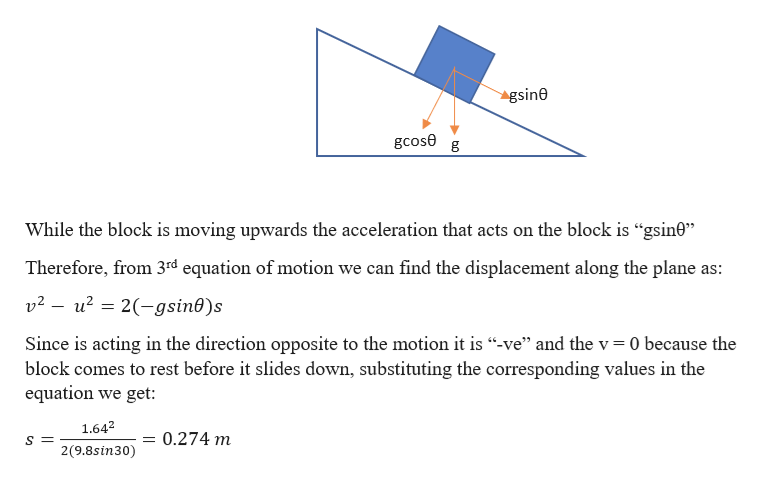# A small block travels up a frictionless incline that is at an angle of 30.0° above the horizontal. The block has speed 1.64 m/s at the bottom of the incline. Assumeg = 9.80 m/s2.How far up the incline (measured parallel to the surface of the incline) does the block travel before it starts to slide back down?

Question

A small block travels up a frictionless incline that is at an angle of 30.0° above the horizontal. The block has speed 1.64 m/s at the bottom of the incline. Assume

g = 9.80 m/s2.

How far up the incline (measured parallel to the surface of the incline) does the block travel before it starts to slide back down?

check_circle

star
star
star
star
star
1 Rating
Step 1

Given information:

Initial velocity of the block (u) = 1.64 m/s

Inclination of the surface (θ) = 300

Acceleration due to gravity (g) = 9.8 m/s2

The distance it travels up the slope (s) =?

Step 2

The situation can be ...help_outlineImage Transcriptionclosegsin0 gcose g While the block is moving upwards the acceleration that acts on the block is "gsin0" Therefore, from 3rd equation of motion we can find the displacement along the plane as: v2-u22-gsin0)s Since is acting in the direction opposite to the motion it is "-ve" and the v 0 because the block comes to rest before it slides down, substituting the corresponding values in the equation we get: 1.642 0.274 m 2(9.8sin30) fullscreen

### Want to see the full answer?

See Solution

#### Want to see this answer and more?

Solutions are written by subject experts who are available 24/7. Questions are typically answered within 1 hour.*

See Solution
*Response times may vary by subject and question.
Tagged in

### Physics